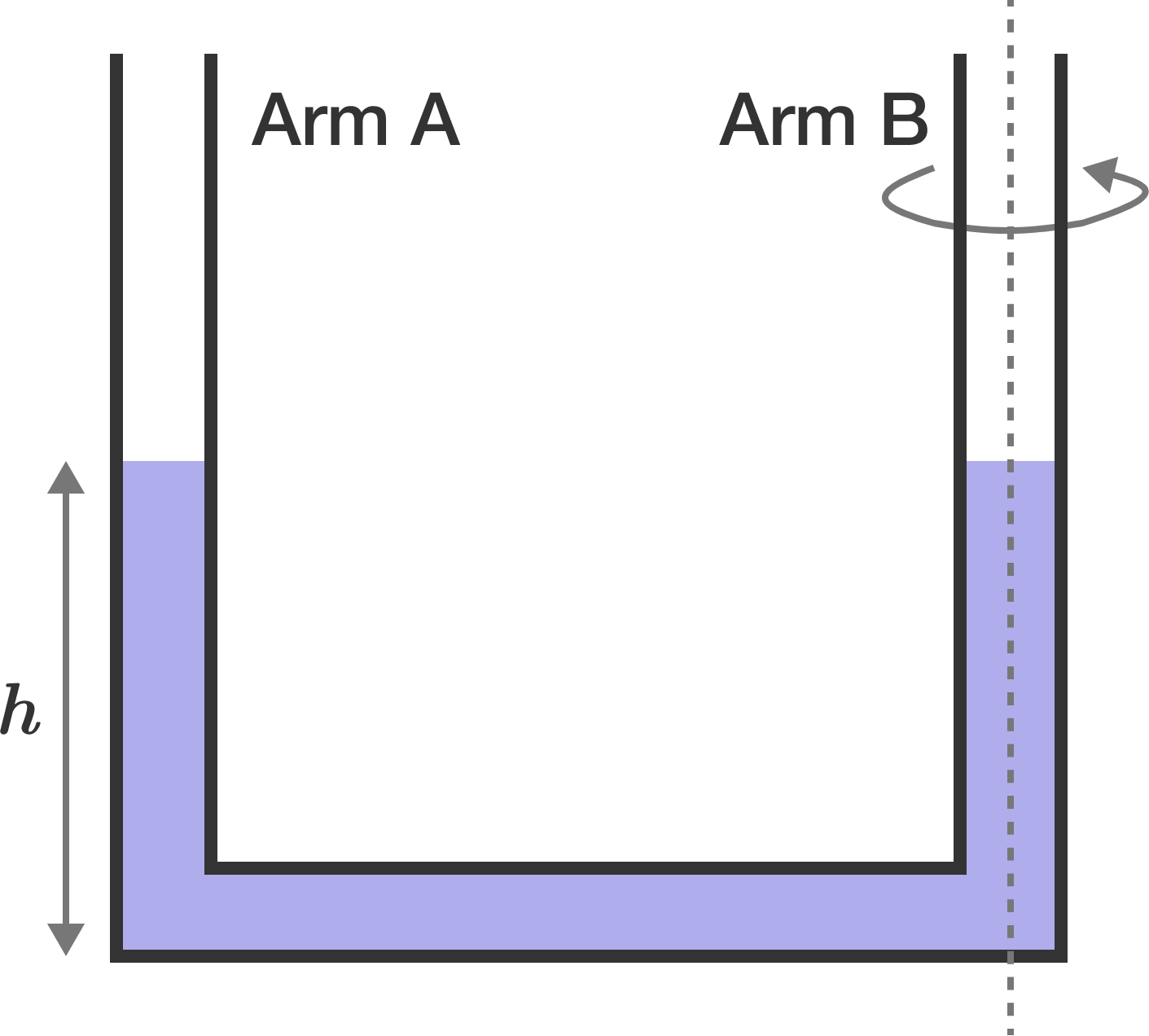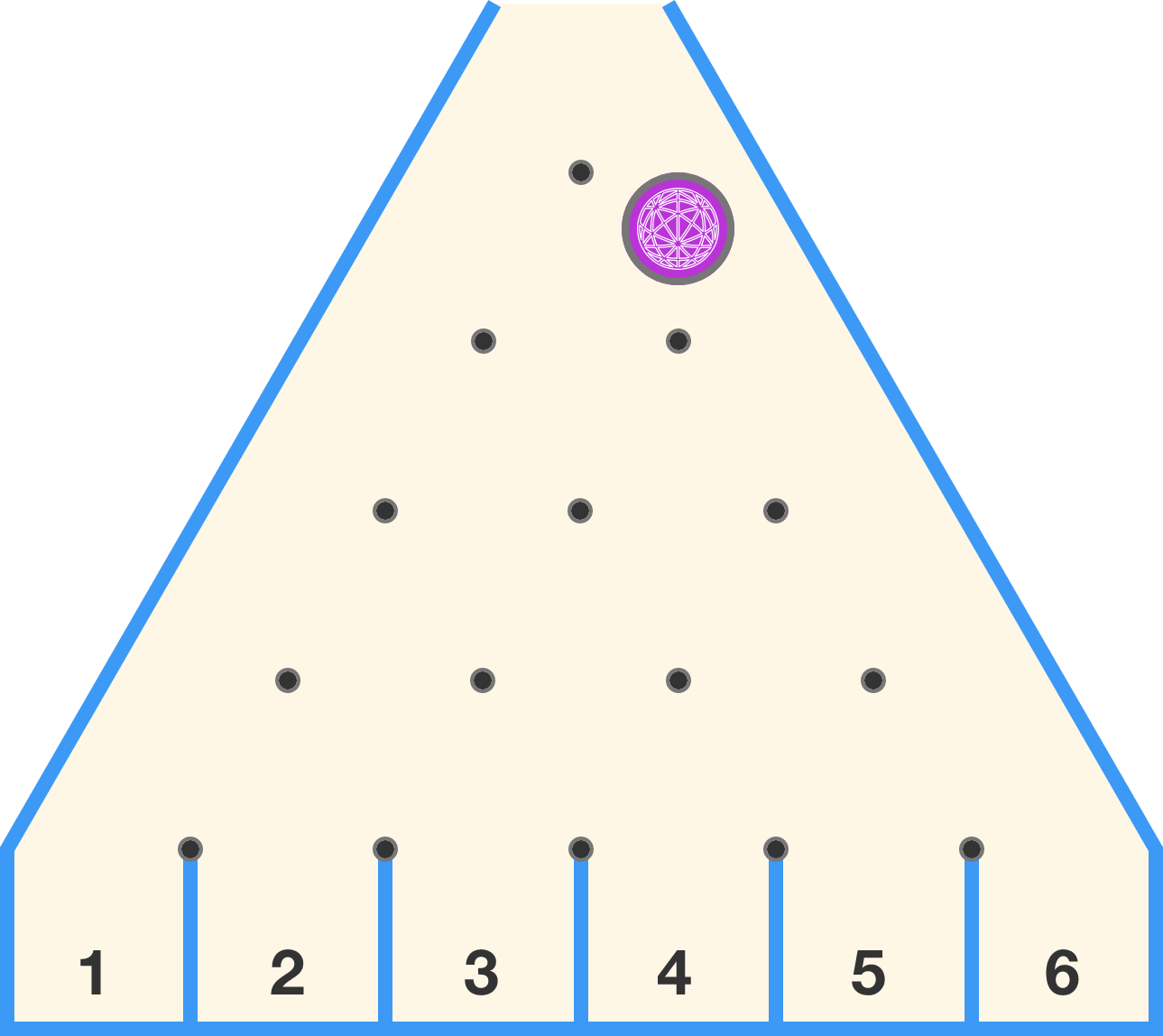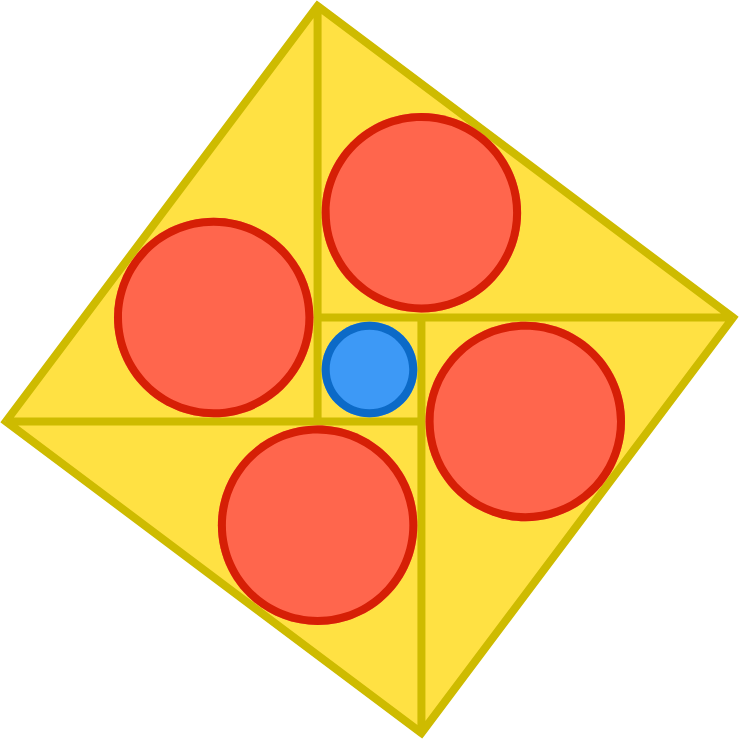# Problems of the Week

Contribute a problem

# 2017-12-04 Basic

Question 1: Are both "yes" and "no" invalid answers to question 2?

Question 2: Is either "yes" or "no" a valid answer to question 1?

What is the answer to Question 2?

Note: Assume the standard laws of logic. If there are no consistent "yes"/"no" answers that satisfy this system, then this is an inconsistent scenario.A U-tube is filled with water to height $h,$ as shown. Then the U-tube is spun at a constant rate about arm B.

What will happen to the water level in arm A?

Four true facts:

$123456789$ is divisible by 3.
$12345+6789$ is divisible by 3.
$123+456+789$ is divisible by 3.
$12+3456+78+9$ is divisible by 3.

True or False?

No matter where and how many $+$ signs you put in between the digits of the number 123456789, the resulting number is still divisible by 3.The figure to the right is a peg board used for a game show. Whenever the purple chip falls onto a peg, it has an equal chance to bounce left or right.

If the chip is dropped from the location shown, which position would it most likely fall to?The blue circle has a radius of length 1.
The four red circles each have a radius of length 2.

What is the side length of the large, outer square?

×